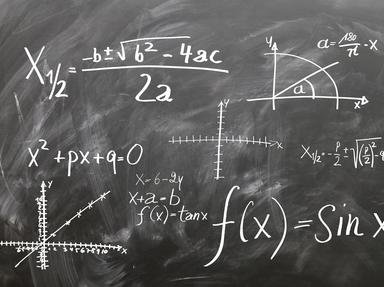# All About Math Trivia Quiz

### This is my first quiz. This is also for kids and for also high school. Some questions are PEMDAS (BEMDAS & BOMDAS to other countries). Enjoy.

A multiple-choice quiz by rjtseelkadoom. Estimated time: 3 mins.

Time
3 mins
Type
Multiple Choice
Quiz #
346,270
Updated
Dec 03 21
# Qns
10
Difficulty
Average
Avg Score
7 / 10
Plays
592
Last 3 plays: Guest 68 (6/10), Guest 174 (7/10), Guest 91 (9/10).
1. PEMDAS (BEMDAS & BOMDAS to other countries): * means multiply and / means divide) : What is (2*90)+(3*20)-100/2? Hint

180
190
170
160

#### NEXT>

2. EXPONENT: ^ means exponent : What is 25^2-199-500?
Hint

74
-64
-74
-84

#### NEXT>

3. WHOLE NUMBERS: It is the least number that is divisible by numbers from 1-10. What is the number? Hint

5040
20160
2550
2520

#### NEXT>

4. PLACE VALUE: The number is 9 876 549. What is the ratio of place value between the bigger 9 (the first 9) and the smaller 9 (the last digit)? Hint

100 000
10 000 000
1 000 000
10 000

#### NEXT>

5. ALGEBRA: What is x in ((2*14+25-10-1)/2)^x=441 Hint

1
4
3
2

#### NEXT>

6. ALGEBRA: What is x if (x^3)-100 is equal to a number that is the median of numbers from 15520 to 15530. Hint

20
35
19
25

#### NEXT>

7. POLYNOMIAL: What is x and y in 7x-5y=100 and 2x+5y=80? Hint

x=30, y=8
y=20, x=8
x=8, y=20
x=20 and y=8

#### NEXT>

8. PEMDAS (BEMDAS & BOMDAS to other countries): What is (3*5)^2-100-99? Hint

126
36
16
26

#### NEXT>

9. PEMDAS: (BEMDAS & BOMDAS to other countries): What is ((2*5+1-9)^6)-100? Hint

36
-46
-26
-36

#### NEXT>

10. INTEGERS: What is (5^2-6)*11-99? Hint

120
130
110
100

 (Optional) Create a Free FunTrivia ID to save the points you are about to earn:Select a User ID:Choose a Password:Your Email:

Quiz Answer Key and Fun Facts
1. PEMDAS (BEMDAS & BOMDAS to other countries): * means multiply and / means divide) : What is (2*90)+(3*20)-100/2?

First you must multiply 2 by 90, and 3 by 20 , then divide 100 by 2, then add the result then subtract it.

Q: 2*90+3*20-100/2
= 180+60-50
= 240-50
= 190
2. EXPONENT: ^ means exponent : What is 25^2-199-500?

Multiply 25 by itself 2 times. Then subtract.

Q: 25^2-199-500
= 625-699
= -74
3. WHOLE NUMBERS: It is the least number that is divisible by numbers from 1-10. What is the number?

Let's start with 1*2*3*4*5*6*7*8*9*10 which is certainly divisible by all of them, but it's not the lowest number.

If the answer is divisible by 10 then it is divisible by 2 and 5.
If the answer it's divisible by 9 then it is divisible by 3.
If it's divisible by 8 then it is divisible by 2 and 4.
If it's divisible by 8 & 9 then it is divisible by 6.

Remove all the duplicates and the lowest number is:
9 x 8 x 7 x 5
= 2520
4. PLACE VALUE: The number is 9 876 549. What is the ratio of place value between the bigger 9 (the first 9) and the smaller 9 (the last digit)?

You must get the value of bigger 9 and the smaller 9. Then divide the value of the bigger 9 to the smaller 9.
5. ALGEBRA: What is x in ((2*14+25-10-1)/2)^x=441

Simplify the inside the parenthisis then reverse the equation.

Q:((2*14+25-10-1)/2)^x=441
= ((28+25-11)/2)^x=441
= ((42)/2)^x=441
= 21^x=441

x=2 (21 squared = 441)
6. ALGEBRA: What is x if (x^3)-100 is equal to a number that is the median of numbers from 15520 to 15530.

First get the median of numbers from 15520 to 15530 and then add 100 and then get the cube root.

median=15525
+100=15625
25^3=15625
7. POLYNOMIAL: What is x and y in 7x-5y=100 and 2x+5y=80?

Add the 2 equations then do the rules of algebra.

2x+5y=80
2x=80-5y
x=40-5/2y

7x-5y=100
7(40-5/2y)-5y=100
280-35/2y-5y=100
560-35y-10y=200
560-200=35y+10y
360=45y
8=y

x=40-5/2y
x=40-5/2*8
x=40-20
x=20
8. PEMDAS (BEMDAS & BOMDAS to other countries): What is (3*5)^2-100-99?

First you must multiply 3 by 5, then multiply the result by itself 2 times, then subtract it in 100 and 99.
Q:(3*5)^2-100-99
= 15^2 -199
= 225-199
= 26
9. PEMDAS: (BEMDAS & BOMDAS to other countries): What is ((2*5+1-9)^6)-100?

First you must do the inside of the parenthesis (aka inside the brackets) then subtract. Since the result is 64, change the equation into 64+(-100).
Q:((2*5+1-9)^6)-100
= ((10+1-9)^6)-100
= 2^6-100
= 64-100
= -36
10. INTEGERS: What is (5^2-6)*11-99?

First you must do the inside the parenthesis (inside the brackets) and then multiply then subtract.
Q:(5^2-6)*11-99
= (25-6)*11-99
= 19*11-99
= 209 -99
= 110